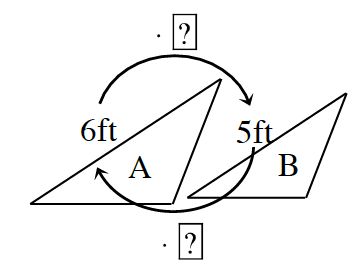### Home > ACC7 > Chapter 7 Unit 4 > Lesson CC2: 7.1.2 > Problem7-22

7-22.

Use the triangles below right to answer the questions that follow.

1. What is the scale factor from A to B?

What scale factor value times $6$ ft would result in $5$ ft? Consider the variable equation with $x$ as the scale factor:
$6x=5$
After isolating $x$, what do you get as the scale factor?

$\frac{5}{6}$

2. What is the scale factor from B to A?

If the change from B to A is just the reverse of the change from A to B, what would the scale factor be? Create an expression like above if necessary.

3. What is the relationship of the scale factors?

The scale factors are reciprocals.